Final Race Results ~ 1999

The 1st section describes all abbreviations used on this page.  The 2nd section lists my placings for each race (WSMC and AHRMA) competed in.  The 3rd section lists my overall WSMC points standings after each race weekend throughout the year in each class..  Click HERE to go to 1998 results, HERE to go to 1997 results.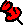1999 WSMC Championship Standings:  (FINAL)

 WSMC Overall Track Championship: 19th WSMC ~ 500 Singles: 3rd WSMC ~ Formula Singles: 4th WSMC ~ Lightweight Vintage: 4th

1999 was another banner year for me ~ 42 race starts, (5) 1st place finishes, (5) 2nd place finishes, (6) 3rd place finishes, (25) top 10 finishes and only (1) DNS due to a blown motor during raceday practice.  I got to compete in the WERA 24 Hour Endurance race at Willow Springs where my team (Waveline Motorsports) and I netted 3rd place in Mediumweight Super Stock and 12th Overall after 722 laps.  I ended up 19th Overall scoring 293 points for the season in the WSMC club, 3rd in 500 Singles (88 pts), 4th in Formula Singles (I actually tied for 3rd with 101 pts but the other guy had more wins) and 4th in Lightweight Vintage (104 pts).  A good year indeed!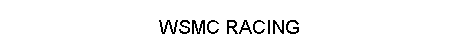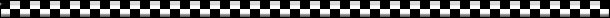Classes will be abbreviated as follows:
FS = Formula Singles
500 = 500 Singles
V = Lightweight Vintage Dinosaurs
SS = 750 Super Stock
MP = 750 Modified Production
SB = 750 Super Bike
F1 = Formula 1
SM = Super Mono 1
SM2 = Super Mono 2
O/A = WSMC Over All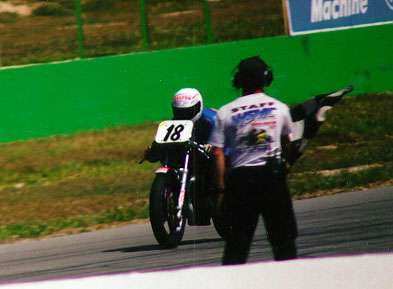The following are my placings for each & all races entered:

WSMC rnd 1:  500=2nd, FS=2nd, V=5th
WSMC rnd 2: 500=3rd, FS=4th, V=7th
WSMC rnd 3: 500=2nd, FS=3rd, V=6th
AHRMA~WSIR, (sat) SM2=1st, SM1=4th
AHRMA~WSIR, (sun) SM2=1st, SM1=4th
WSMC rnd 4:  500=1st, FS=1st, V=2nd
AHRMA~Sears Pt, (sat)  SM2=1st, SM1=6th
AHRMA~Sears Pt, (sun) SM2=2nd, SM1=7th
WSMC rnd 5: DNS ~ blown motor in practice
WSMC rnd 6:  500=3rd, FS=5th, V=6th
WSMC rnd 7:  500=3rd, FS=4th, V=6th
WSMC rnd 8:  500=4th, FS=5th, V=8th
WSMC rnd 9:  500=4th, FS=6th, V=8th
WERA 24 hr Endurance ~ MidWt SS=3rd, O/A=12th!!!
WSMC rnd 10: 500=4th, FS=8th, V=5th
WSMC rnd 11: 500=4th, FS=5th, V=4th
WSMC rnd 12: 500=3rd, FS=8th, V=5thThe following are my individual class standings & WSMC Overall after each event:

WSMC rnd 1:  500=2nd, FS=2nd, V=5th, O/A=26th
WSMC rnd 2: 500=2nd, FS=2nd, V=5th, O/A=18th
WSMC rnd 3: 500=2nd, FS=3rd, V=4th, O/A=24th
WSMC rnd 4:  500=1st, FS=2nd, V=4th, O/A=15th
WSMC rnd 5:  500=2nd, FS=2nd, V=4th, O/A=23rd
WSMC rnd 6:  500=2nd, FS=3rd, V=4th, O/A=24th
WSMC rnd 7:  500=3rd, FS=3rd, V=5th, O/A=24th
WSMC rnd 8: 500=3rd, FS=3rd, V=5th, O/A=24th
WSMC rnd 9:  500=3rd, FS=3rd, V=5th, O/A=22nd
WSMC rnd 10: 500=3rd, FS=3rd, V=5th, O/A=24th
WSMC rnd 11: 500=3rd, FS=3rd, V=4th, O/A=21st
WSMC rnd 12: 500=3rd, FS=4th, V=4th, O/A=19th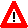All images, pictures & information contained within this website
property of &